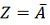# NOT Operation

Like OR and AND operation, the NOT Operation is also an important function in the digital electronic circuit. It is denoted by a bar. The binary not operation is also known as not function or complement function or bit invert function.

The not operation has only one input and one output. The input and output of the not operation are either zero or one. In the negative logic, 0 stands for high and 1 stands for low signals. The not operation will always produce a 0 out if their input is 1 and if their input is 0 then it produces the output, 1.Their operation is analogous to a negative operation which has single input and output. This operation is explained by the example shown below:

Example: Consider the word statement  Z = A Not A. In boolean algebra it is expressed as:For the given operation the output can be expressed as:The NOT operation is an inversion as shown below by the truth table. It is read as “ not x is equal to z”.

xz
01
10

When the value of x is equal to 0 the value of z is equal to 1. When x is equal to 1 the value of z is equal to 0.

Also See:: AND Operation

Also See: OR Operation